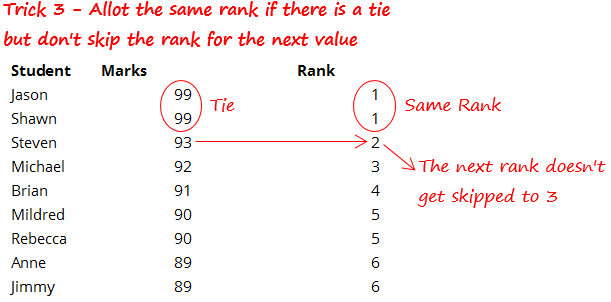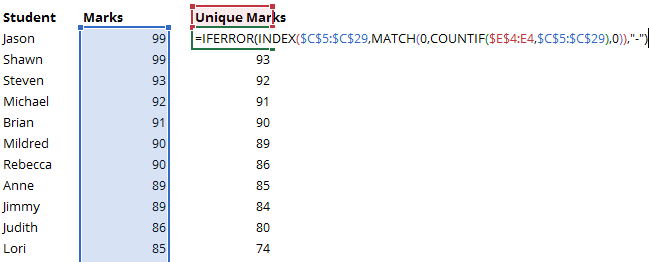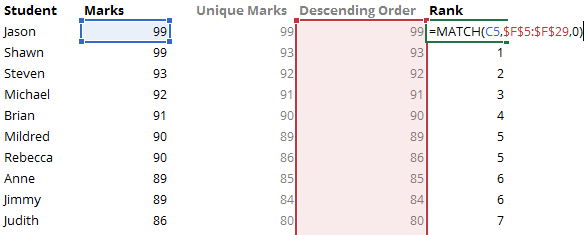Ranking is one of the very common aspects of data analysis. In this post you’ll not only learn how to rank data in excel but also apply some variations to your ranking criteria.

Let take a look 3 tricks for ranking data in Excel

## Let’s begin with this data !Note that as of now our data is sorted in descending order but our formulas will work even for unsorted data

## Trick 1) Skip the next rank when there is a tieHow do we do this – by using the simple RANK function. Take a lookThe Rank Function accepts 3 Inputs

1. Number – Which number do you want to rank
2. Reference – List of numbers
3. Order – 0 Stands for descending and 1 stands for ascending. By defaults excel picks up 0

The Rank function will automatically award the same rank to the ties and skip the rank for the largest next value.

The newer versions of Excel have the new RANK.EQ function which is exactly the same as RANK function. The only difference is that it is compatible with the newer version of Excel

## Trick 2) Allot ranks in a serial order even if there is a tieFor doing this we need to add a COUNTIF function along with the RANK Function1. The rank is doing the same work as seen in Trick 1
2. But the COUNTIF is checking that if the number has appeared in the range previous to the current cell or not
3. And adding that count to the current rank

## Trick 3) Same rank incase a tie but don’t skip rank for the next valueThis one gets a bit tricky. Let’s solve this in 3 Steps

Step 1) Find the Unique Marks (i.e. none of the marks get repeated).1. Note that this is an array formula and you must confirm the formula by pressing CTRL + SHIFT + ENTER and not the usual Enter key
2. Related – How to extract unique values from a range

Step 2 – Arrange the unique marks in descending order (largest to smallest)1. As of now it might look stupid to arrange the data in descending order since it is already in that order
2. But remember the data could be in any order so I am adding this additional step
3. I have used the LARGE and the ROWS formula here

Step 3 – Find the Rank using Match Function1. The Match function will return the same rank for ties
2. And the next rank (without skipping) for the next largest value